# Area of Trapezoid

Recall that the area of a triangle is equal to half of the base times the height.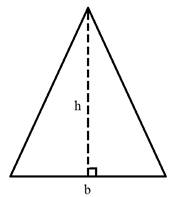Now, let's look at what would happen if we cut the top off of the triangle.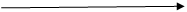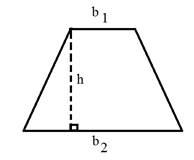The new shape is a trapezoid. A trapezoid is a quadrilateral with only one pair of opposite sides that are parallel. We must take the average of the bases and then multiply by the height. That means that we need to add the bases together and divide by 2. Then we will multiply by the height.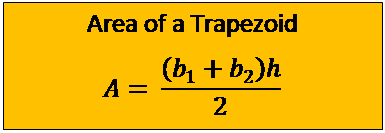Determine the area of the following trapezoids.

1.)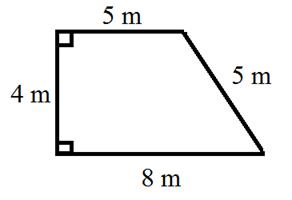Be sure to use the parallel sides as the bases and the height is the piece that makes a right angle with the parallel sides.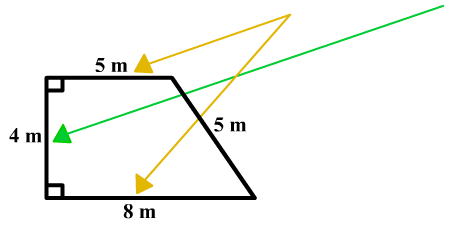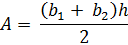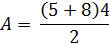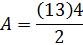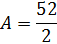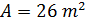2.)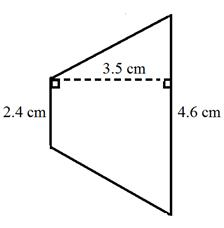This trapezoid is on its side. The height connects the two bases.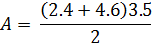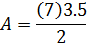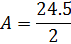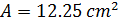3. The area of the trapezoid is 168 in2. Determine the height.In this example, the area has been given, so we will work backwards to determine the height.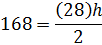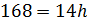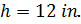Notice that in this example, the height is labeled in inches and not inches squared. The height is one dimensional, whereas the area is two dimensional. Therefore, the height is labeled inches where the area is labeled square inches.

Let's Review

To determine the area of a trapezoid follow three steps.

2.) Multiply the sum by the height.

3.) Divide the product by 2.

4.) Be sure the label the area with "units squared."

 Related Links: Area of a Trapezoid Worksheets Math Geometry Area of Trapezoid Perimeter of Trapezoid